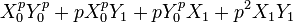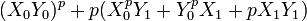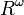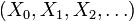# Ring of Witt vectors

## Definition

The term ring of Witt vectors or Witt ring is a commutative unital ring defined in the context of a prime number$p$ and a commutative unital ring$R$. There are two version of this:

• The ring of Witt vectors, by default, refers to infinite sequences, i.e., Witt vectors of infinite length (the detailed definition is given below).
• The ring of Witt vectors of length$d$ refers to the quotient of this ring obtained by simply looking at the first$d$ coordinates of the vector. The operations are defined in a manner that the first$d$ coordinates of the sum and product depend only on the first$d$ coordinates of the vectors being added or multiplied. Thus, it is legitimate to pass to quotients in this manner.

There is a related notion called the ring of universal Witt vectors that makes sense in the context of any commutative unital ring$R$ and does not require specification of any prime number.

### Definition of the ring of Witt vectors of infinite length

We first define the Witt polynomials as the following polynomials in$\mathbb{Z}[X_0,X_1,X_2,\dots,]$ (and hence interpretable in$R[X_0,X_1,X_2,\dots,]$):$W_n(X) := \sum_{i=0}^n p^iX_i^{p^{n-i}}$

The first few Witt polynomials are given below:$n$$W_n$
0$X_0$
1$X_0^p + pX_1$
2$X_0^{p^2} + pX_1^p + p^2X_2$
3$X_0^{p^3} + pX_1^{p^2} + p^2X_2^p + p^3X_3$

We now consider the set$(X_0,X_1,X_2,\dots,X_n,\dots), X_i \in R$ of all sequences over$R$. We call the elements of this set Witt vectors, and we define the addition and multiplication as follows:

Addition and multiplication are defined in the unique manner so as to make the set of Witt vectors a commutative unital ring with the following two properties: (a) each Witt polynomial is a ring homomorphism from that ring to$R$, and (b) both addition and multiplication are given by polynomials with integer coefficients that depend on$p$ but not on$R$.

Explicitly, the sum and product of the Witt vectors$(X_0,X_1,X_2,\dots,)$ and$(Y_0,Y_1,Y_2,\dots,)$ are given by the following formulas:$n$$n^{th}$ coordinate of sum$n^{th}$ coordinate of product
0$X_0 + Y_0$$X_0Y_0$
1$X_1 + Y_1 - \sum_{i=1}^{p-1} \frac{(p-1)!}{i!(p-i)!} X_0^iY_0^{p-i}$, which can be thought of as$X_1 + Y_1 + \frac{(X_0^p + Y_0^p) - (X_0 + Y_0)^p}{p}$ (although the latter notation does not directly make sense unless$p$ is invertible in$R$).$X_0^pY_1 + Y_0^pX_1 + pX_1Y_1$

Some elaboration on why these choices work is provided below:$n$$W_n(X)$$W_n(Y)$$W_n(X) + W_n(Y)$, which equals$W_n$ of the Witt sum$W_n(X)W_n(Y)$, which equals$W_n$ of the Witt product Comment
0$X_0$$Y_0$$X_0 + Y_0$$X_0Y_0$ Zeroth Witt polynomial is projection onto zeroth coordinate; pointwise operations mean this is a homomorphism.
1$X_0^p + pX_1$$Y_0^p + pY_1$$X_0^p + Y_0^p + p(X_1 + Y_1)$, which is the same as$(X_0 + Y_0)^p + p\left[X_1 + Y_1 + \frac{(X_0^p + Y_0^p) - (X_0 + Y_0)^p}{p}\right]$$X_0^pY_0^p + pX_0^pY_1 + pY_0^pX_1 + p^2X_1Y_1$, which is the same as$(X_0Y_0)^p + p(X_0^pY_1 + Y_0^pX_1 + pX_1Y_1)$ Things work out.

### Truncations to finite length

We can also consider the ring of Witt vectors of length$d$. This is obtained by taking the ring of Witt vectors and projecting it to the first$d$ coordinates$X_0,X_1,X_2,\dots,X_{d-1}$. The operations all have the property that the formula of the$i^{th}$ coordinate of the sum and product involves only vectors$X_j,Y_j, j \le i$, so the ring operations descend to the quotient and we get a ring structure on the quotient.

## Particular cases

Note that$p$ is the prime with respect to which we are defining the Witt polynomials.

Condition on$R$ Conclusion for ring of Witt vectors (infinite) Conclusion for truncation to length$d$$p$ is invertible in$R$ Isomorphic to the external direct product of countably many copies of$R$ (i.e., sequences with pointwise sum and product), i.e.,$R^{\omega}$ Isomorphic to$R^d$$R$ is the finite prime field$\mathbb{F}_p$ Isomorphic to the ring of p-adic integers, where a Witt vector$(X_0,X_1,X_2,\dots)$ is identified with the$p$-adic integer$\sum_{i=0}^\infty X_ip^i$. Isomorphic to the ring of integers mod$p^d$, i.e., the ring$\mathbb{Z}/p^d\mathbb{Z}$.$R$ is a finite field of order$p^k$,$k$ a natural number Isomorphic to an unramified degree$k$ extension of the ring of p-adic integers. There is only one such extension up to isomorphism. Isomorphic to the Galois ring, which is an unramified extension of$\mathbb{Z}/p^d\mathbb{Z}$ of degree$k$, and can be constructed using any minimal polynomial for$\mathbb{F}_{p^k}$ over$\mathbb{F}_p$.